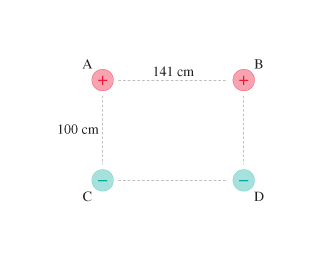# Problem: The figure shows four electrical charges located at the corners of a rectangle. Like charges, you will recall, repel each other while opposite charges attract. Charge B exerts a repulsive force (directly away from B) on charge A of 3.6 N . Charge C exerts an attractive force (directly toward C) on charge A of 7.2 N . Finally, charge D exerts an attractive force of 2.4 N on charge A.Assuming that forces are vectors, what is the magnitude of the net force net exerted on charge A?What is the direction of the net force net exerted on charge A?

###### Problem Details

The figureshows four electrical charges located at the corners of a rectangle. Like charges, you will recall, repel each other while opposite charges attract. Charge B exerts a repulsive force (directly away from B) on charge A of 3.6 N . Charge C exerts an attractive force (directly toward C) on charge A of 7.2 N . Finally, charge D exerts an attractive force of 2.4 N on charge A.

Assuming that forces are vectors, what is the magnitude of the net force net exerted on charge A?

What is the direction of the net force net exerted on charge A?